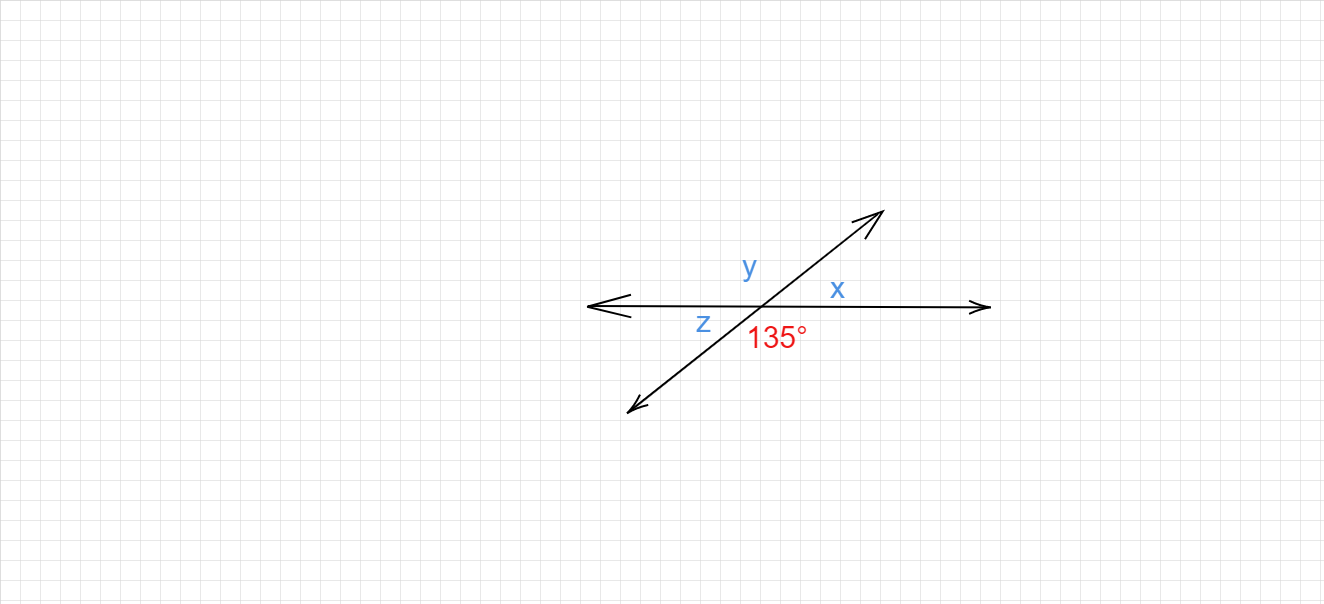"
">

# Find the values of x , y and z."

Given :

The figure of two intersecting lines is given.

To do :

We have to find the values of x , y and z.

Solution :

We know that,

Vertically opposite angles are equal.

Sum of the angles on a straight line is $180°$.

In the given figure,

$135°$ and y are vertically opposite angles.

Therefore,

$y = 135°$

$135°$ and x lie on a straight line.

This implies,

$x+135° = 180°$

$x = 180°-135°$

$x = 45°$

$z = x = 45°$   (vertically opposite angles).

Updated on: 10-Oct-2022

27 Views